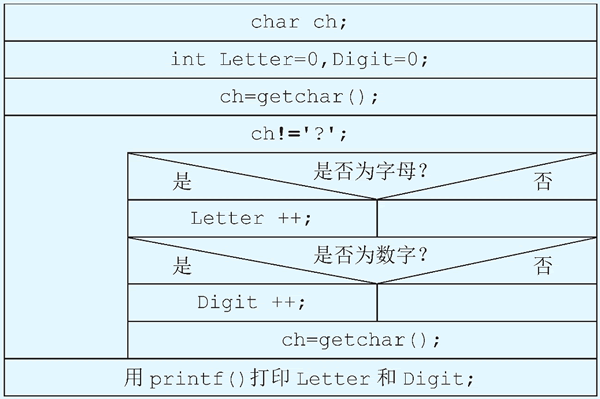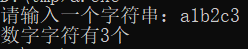• ## c语言统计字符个数

万次阅读 2019-05-21 23:00:01
1.统计字符串出现的次数 int main(int argc, const char * argv[]) { char chs[] = "fjadsjfjdsajfkjdskj"; int arr = {0};//26字母 for (int i = 0; i < sizeof(chs); i++) { if(*(chs+i)){ ...
1.统计各字符串出现的次数
int main(int argc, const char * argv[]) {
char chs[] = "fjadsjfjdsajfkjdskj";
int arr = {0};//26个字母
for (int i = 0; i < sizeof(chs); i++) {
if(*(chs+i)){
arr[*(chs+i) - 'a']++;
}

}
for (int j = 0; j < sizeof(arr)/sizeof(int); j++) {
if(arr[j]!=0){
printf("%c = %d\n",'a'+j,arr[j]);
}

}
return 0;
}



展开全文• 本文给大家分享的是2则C语言实现统计字符个数的代码，非常的简单实用，小伙伴们根据自己的项目需求自由选择吧。
• 这是一个计数问题：重复读入字符，根据字符的类型(字母还是数字)，进行个数统计。但是，并不知道输入了多少个字符，只知道输入的字符串中最后一个字符是?。因此，可以使用 while 语句，当读入的字符是?时结束循环，...
这是一个C语言 while 循环示例：输入一串以?为结束标志的字符，统计其中字母和数字的个数。问题分析输入：从键盘输入一个以?结尾的字符串。输出：两个整数(分别表示字母和数字的个数)。这是一个计数问题：重复读入字符，根据字符的类型(字母还是数字)，进行个数统计。但是，并不知道输入了多少个字符，只知道输入的字符串中最后一个字符是?。因此，可以使用 while 语句，当读入的字符是?时结束循环，否则判断读入的字符是字母还是数字，并累加计数。那么，如何判断一个字符是字母还是数字呢？计算机中所有的字符都是以数字的形式存在的，每一个字符都有一个数字与其对应(参见表 ASCII 标准字符代码表)。因此，一个字符变量“ch”的值是字母还是数字，可以用下面的条件表达式进行判断：字母：((ch >= 'a') && (ch <= 'z')) || ((ch >= 'A') && (ch <= 'Z'))数字：(ch >= '0') && (ch <= '9')算法描述1) 定义字符型变量 ch 用来存放一个字符；2) 定义整型变量 Letter 和 Digit，作为计数器，分别存放字母和数字的个数，并初始化为 0；3) 当 ch 不是“？”时，重复执行：若 ch 是字母，则字母个数 Letter 加 1；若 ch 是数字，则数字个数 Digit 加 1；读入一个新字符给 ch；4) 输出 Letter 和 Digit；5) 结束。代码清单 1：输入一串以?为结束标志的字符，统计其中字母和数字的个数#include #include int main( ){char ch;int Letter = 0,Digit = 0;printf("输入一串‘?’结尾的字符：");ch = getchar( );                                   //循环外读入第一个字符while(ch != '?'){if(((ch >= 'a') && (ch <= 'z')) || ((ch >= 'A') && (ch <= 'Z')))Letter++;                                  //字母的个数加 1else if((ch >= '0') && (ch <= '9'))Digit++;                                   //数字的个数加 1ch = getchar( );                               //继续读入下一个字符}printf("其中字母的个数是：%d\n",Letter);printf("其中数字的个数是：%d\n",Digit);system("pause");return 0;}运行结果：输入一串‘?’结尾的字符：321Are you ready?其中字母的个数是：11其中数字的个数是：3
展开全文• 统计字符数 [问题描述] 给定-一由a-z这26字符组成的字符串,统计其中哪个字符出现的次数最多。 [输入格式] 输入包含一行,一字符串,长度不超过1000。 [输出格式] 输出一行,包括出现次数最多的字符和该字符出现的...
统计字符数
[问题描述]
给定-一个由a-z这26个字符组成的字符串,统计其中哪个字符出现的次数最多。
[输入格式]
输入包含一行,一个字符串,长度不超过1000。
[输出格式]
输出一行,包括出现次数最多的字符和该字符出现的次数,中间以一个空格分开。如果有多个字符出现的次数相同且最多,那么输出ascii码最小的那一个字符。[输入样例]
abbccc
[输出样例]
c 6
#include<stdio.h>//字母次数
#include<string.h>
int main()
{
char str,ch;
int a={0},b,c;
scanf("%s",str);
int i,j,k;
for(i=0;i<strlen(str);i++)
{
b=str[i];
a[b]++;
}
int MAX=a;
for(i=0;i<150;i++)
{
if(a[i]>MAX) MAX=a[i],c=i;
}
ch=c;
printf("%c %d",ch,MAX);
return 0;
}

或者
#include<stdio.h>
#include<string.h>
int maxvalue(int *value,int index)
{
int i;
int j;
int max = 0;
for(i=0;i<index-1;i++){
for(j=i+1;j<index;j++){
if(value[i] <= value[j]){
if(max < value[j]){
max = value[j];
}
}
}
}
return max;
}

int main()
{
char buff;
int count = {0};
int i=0;
int max_count;
memset(buff,0,1024);
scanf("%s",buff);
getchar();
while(buff[i] != '\0'){
if(buff[i] >= 'a' && buff[i] <= 'z'){
count[buff[i] - 'a']++;
}
i++;
}
max_count = maxvalue(count,26);
for(i = 0;i < 26;i++){
if(count[i] == max_count){
printf("%c %d",'a'+i,count[i]);
}
}
}




展开全文• #include<... printf("请输入一个字符串："); gets(s); for(i=0;i<strlen(s);i++){ if(s[i]>='0'&&s[i]<='9'){ count++; } } printf("数字字符有%d",count); } ...

方法一：常规计算

#include<stdio.h>
#include<string.h>
void main(){
int i,count=0;
int length=0;
char s;
printf("请输入一个字符串：");
gets(s);
length=strlen(s);
for(i=0;i<length;i++){
if(s[i]>='0'&&s[i]<='9'){
count++;
}
}
printf("数字字符有%d个",count);
}



方法二：函数调用

#include<stdio.h>
#include<string.h>
void string_num(char s[]){
int i,length=0,count=0;
length=strlen(s);
for(i=0;i<length;i++){
if(s[i]>='0'&&s[i]<='9'){
count++;
}
}
printf("数字字符有%d个",count);
}
void main(){
int i,count=0;
int length=0;
char s;
printf("请输入一个字符串：");
gets(s);
string_num(s);
}`
展开全文• 题目：输入一行字符，分别统计出其中的英文大小写字母、空格、数字和其它字符的个数。
• 题目来源：分类统计字符个数 PTA 本题要求实现一个函数，统计给定字符串中英文字母、空格或回车、数字字符和其他字符的个数。 函数接口定义： void StringCount( char s[] ); 其中 char s[] 是用户传入的字符串。...
• C语言统计字符串中单词个数 统计单词，重要的是关注单词的最后一个字母 有两种情况 本身不是空格，而且下一个是空格或者‘\0’结尾标志 此次代码心得：字符串结尾是‘\0’，空格和’\0’不一样 ...
• 题目：统计字符串中单词个数，这里的单词是指除了空格之外的其它一个或者几个组成的字符段。 上代码 #include <stdio.h> int main(){ char a = {0}; int i = 0, sign = 0, num = 0; gets(a); ...
• 统计字符 C语言 PTA 实验3-4 本题要求编写程序，输入10个字符，统计其中英文...letter = 英文字母个数, blank = 空格或回车个数, digit = 数字字符个数, other = 其他字符个数 的格式输出。 输入样例: aZ & 0...
• 满意答案aaatanglei2013.09.30采纳率：42%...而你定义的数组s是字符型的，咱先别管x[s[i]-'a']++这里面的，咱就先看你首先不是不要保证x数组里面的为整数，C语言定义两个字符相加或相减得到的就是整数，即你里面的...
• //用来统计个数。 char str;//存储字符串。 int i; gets(str);//输入字符串。 for(i = 0; str[i]!=’\0’; ++i)//遍历字符串。 cnt[str[i]]++;//统计个数。 for(i=0;i&lt;128; i ++)//遍...C
• 对于给定的一个字符串，统计其中数字字符出现的次数。 #include<stdio.h> int main(void) { /*********Begin*********/ char s; int n; scanf("%d",&n); while(n--) { int count=0; s...
• 程序要求满足以下功能将字符串数组中每一行中的大写字母A、B、C都换成数字2,并且去除字符串中的连字符号‘-’,但是数字保持不变。设字符串数组有3行。例如:字符串内容为:输出的内容为A-BC-CBC222222A-12- 3C-3-1...
• 前天用java写了这功能，今天用C语言实现了一下，有点恼火的就是判断每行字符串最后一个字符了，如果为空格的话就要特别处理一下，还要注意，记得每行时都要将 isLastBlank重置为1，而且刚刚开始时候他的值是为0....
• 本题要求实现一个函数，统计给定...letter = 英文字母个数, blank = 空格或回车个数, digit = 数字字符个数, other = 其他字符个数 的格式输出 裁判测试程序样例： #include <stdio.h> #define MAXS 15 void
• 编写一函数count，有实参传来一个字符串，统计字符串中字母、数字、空格和其他字符的个数，在主函数中输入字符串以及输出上述的结果。 要求：(1)定义统计函数count来实现。 函数的原型为：void count(char str...
• 第一行是测试数据的组n，每组测试数据占1行，是一由a~z这26个字符组成的字符串，每组测试数据之间有一空行，每行数据不超过1000个字符且非空。 Output  n行，每行输出对应一输入，包括出现次数最多的...
• 输入ASCII字符，次数未知，统计输入的字符总数，有哪些字符输入了，每个字符的输入次数，每个字符占总输入字符数的比例，把字符按比例大小从大到小排序。
• /*     题目1：    输入一行字符，分别统计出其中英文字母、空格、数字和其它字符的个数。    我的思路： ... 首先利用if语句写出是什么字符，然后，再用其统计个数，最后在写上到什么时候截止
• 该楼层疑似违规已被系统折叠隐藏此楼查看此楼#includemain(){intacount=0,bcount=0,...printf("请输入一行字符:\n");a=getchar();while(a!='\n'){switch(a){case'q':case'w':case'e':case'r':case't':case'y':case'...
• C语言统计字符串中的各种字符1.输入代码，结果如下。2.源代码 1.输入代码，结果如下。 输入字符串：this is mingfa site,welcom here!统计其中的字母、数字、空格和其他字符的个数。 2.源代码 #include<stdio.h&......

# c语言统计字符个数c语言 订阅